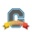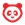cancel
Showing results for
Search instead for
Did you mean:Community Member

## Multi-part question with numerical answers

Jump to solution

I would like to create a multi-part question with numerical answers. I do not see any instructions in the instructor guide about these types of questions. Here is an example.

Question 1

Price per unit = \$10; Cost per unit = \$8; Number of units = 100.

a. Calculate revenue.

b. Calculate cost.

c. Calculate profit.

Furthermore, I would like to assign different points for each part. Thanks.

Tags (2)
1 Solution

Accepted SolutionsCommunity Coach

@mpn ​, there's no way to do this directly in Canvas, but it is possible through the use of multiple questions. Below is an example of how this would look/work. The first version is the Instructor view and the second is how it would look to students.To create this I used a text (no question) type for the initial information and then fill in the blank questions for the actual questions. Here's the link to information on quizzes (and quiz questions) that should help - Quizzes

Hope this helps!

5 RepliesCommunity Team

@mpn ​, the way I would create this is to place the instructions for the three questions in a "Text-No Question" question type, along with a directiive to students that the next three questions will use the information. I would then create three separate questions for the three calculations, with each question carrying the point values you want assigned to it. If you decide to use this method, make sure not to use question groups to randomize your questions, because if you do, the three questions won't immediately follow the "Text-No Question" question containing the instructions.Community Coach

@mpn ​, there's no way to do this directly in Canvas, but it is possible through the use of multiple questions. Below is an example of how this would look/work. The first version is the Instructor view and the second is how it would look to students.To create this I used a text (no question) type for the initial information and then fill in the blank questions for the actual questions. Here's the link to information on quizzes (and quiz questions) that should help - Quizzes

Hope this helps!Community Member

This works to some extent.  I have a follow up question. I tried to title the initial text (no question) "Question 1" and the actual 3 questions "Question 1a", "Question 1b", "Question 1c" which makes it clear to students that the three questions are linked to the initial text. While these titles show up in my Edit view, they do not show up in the Preview. In the Preview they show up as Question 2, Question 3, and Question 4. Another complication is  that under Assignments, it shows as if the quiz has 4 questions (Canvas is counting the initial text as a question) which might confuse students. Any suggestions?Community Coach

Unfortunately there's nothing that can be done about either of your issues. You can't set your own numbers for questions, the best you can do is add a number or A, B, C, etc... to the actual question text. As for the other issue with text (no question) showing up as a question is well known and I'm not aware of a way around it.Surveyor

Seem a bit late.

In Quizzes.next, you can use the "stimulus" question type for multi-part questionsUnanswered Topics